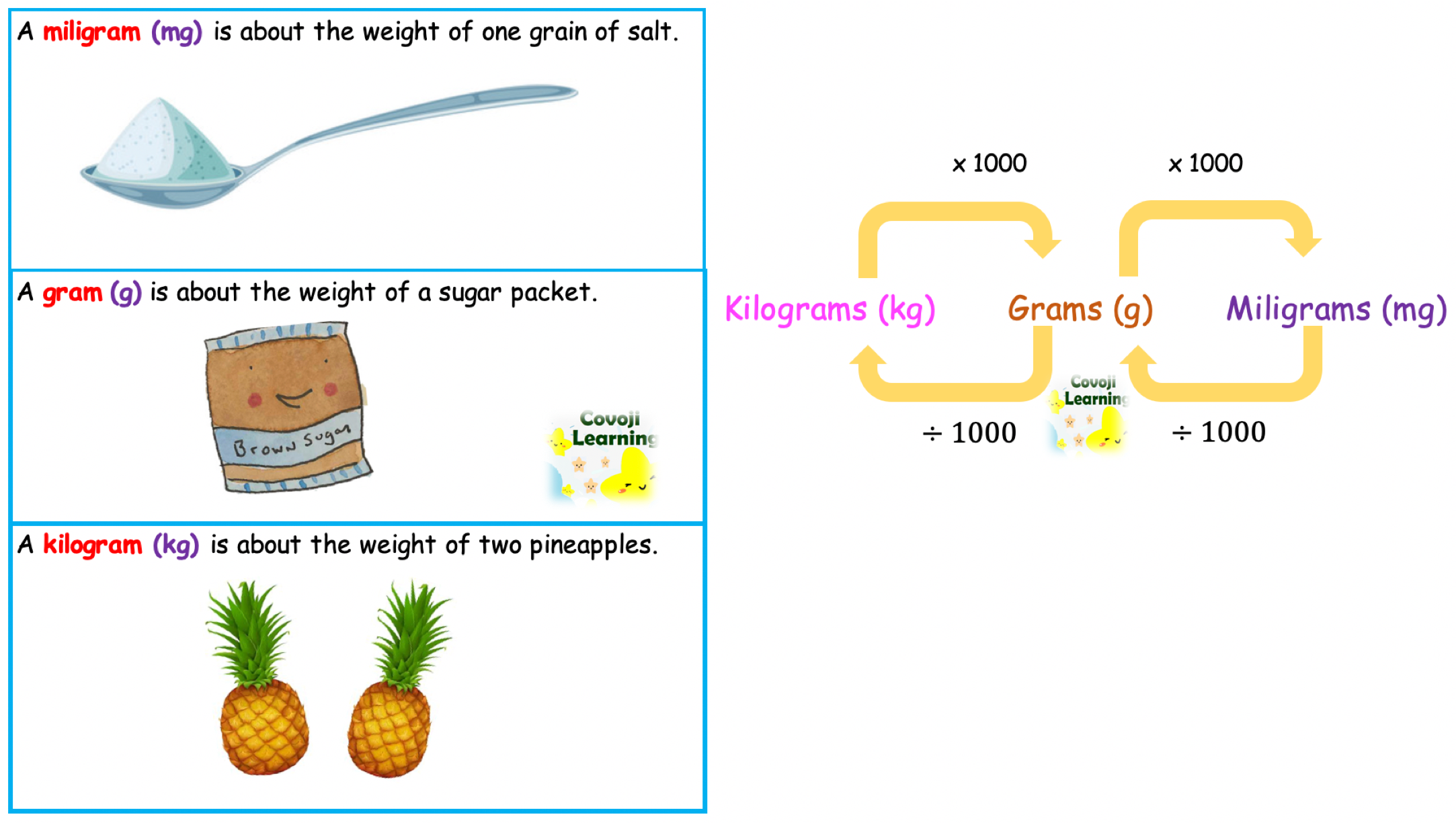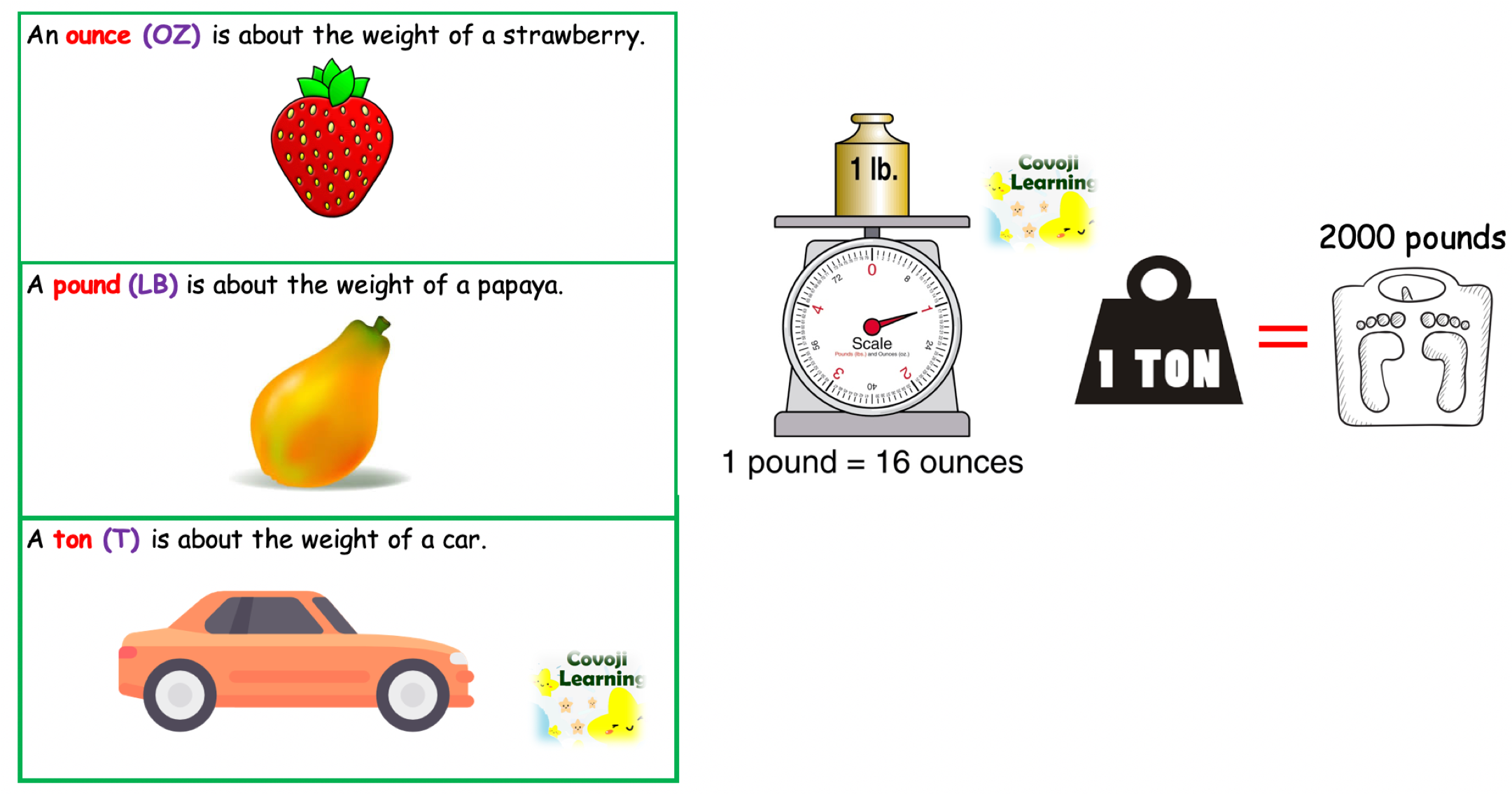Weight is the measure of how heavy an object is. The weight of an object can be measured using different types of weighing scales. These weighing scales use standard units such as milligrams, grams and kilograms to measure the weight of different objects. There are two types of measuring system in the world which are metric system and US customary system.

### Metric Units### US Customary Units### Note:

1. To convert from larger units to smaller units, multiply by the appropriate unit ratio.
2. To convert from smaller units to larger units, divide by the appropriate unit ratio.
Categories: Math Zone